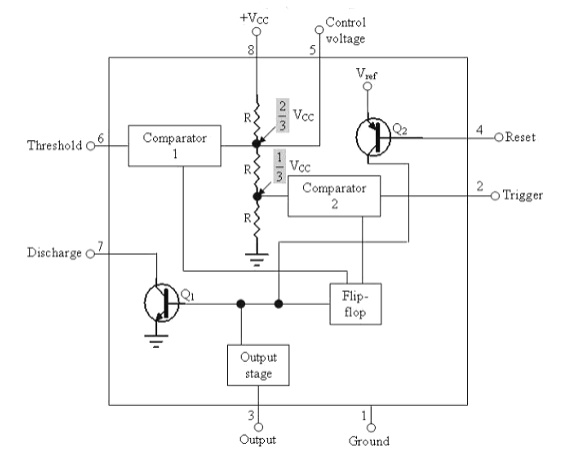# Block Diagram of 555 Timer ICFrom the above figure, three 5k internal resistors act as voltage divider providing bias voltage of 2/3 Vcc to the upper comparator & 1/3 Vcc to the lower comparator.

Block Diagram of 555 Timer IC:From the above figure, three 5k internal resistors act as voltage divider providing bias voltage of 2/3 Vcc to the upper comparator & 1/3 Vcc to the lower comparator. It is possible to vary time electronically by applying a modulation voltage to the control voltage input terminal (5).

(i)       In the Stable state:

The output of the control FF is high. This means that the output is low because of power amplifier which is basically an inverter. Q = 1; Output = 0

(ii)    At the Negative going trigger pulse:

The trigger passes through (Vcc/3) the output of the lower comparator goes high & sets the

FF.Q = 1;  Q = 0

(iii)  At the Positive going trigger pulse: It passes through 2/3Vcc, the output of the upper comparator goes high and resets the FF. Q = 0; Q = 1 The reset input (pin 4) provides a mechanism to reset the FF in a manner which overrides the effect of any instruction coming to FF from lower comparator.

Monostable Operation:Model Graph:Initially when the output is low, i.e. the circuit is in a stable state, transistor Q1 is ON & capacitor C is shorted to ground. The output remains low. During negative going trigger pulse, transistor Q1 is OFF, which releases the short circuit across the external capacitor C & drives the output high. Now the capacitor C starts charging toward Vcc through RA. When the voltage across the capacitor equals 2/3 Vcc, upper comparator switches from low to high. i.e. Q = 0, the transistor Q1 = OFF ; the output is high.Since C is unclamped, voltage across it rises exponentially through R towards Vcc with a time constant RC (fig b) as shown in below. After the time period, the upper comparator resets the FF, i.e. Q = 1, Q1 = ON; the output is low.[i.e discharging the capacitor C to ground potential (fig c)]. The voltage across the capacitor as in fig (b) is given byIf the reset is applied Q2 = OFF, Q1 = ON, timing capacitor C immediately discharged. The output now will be as in figure (d & e). If the reset is released output will still remain low until a negative going trigger pulse is again applied at pin 2.

Study Material, Lecturing Notes, Assignment, Reference, Wiki description explanation, brief detail

Related Topics